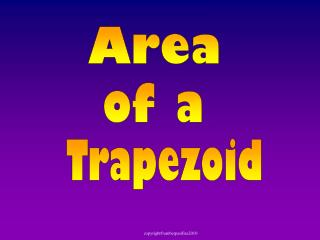DownloadDownload PresentationArea

# Area

Download Presentation## Area

- - - - - - - - - - - - - - - - - - - - - - - - - - - E N D - - - - - - - - - - - - - - - - - - - - - - - - - - -
##### Presentation Transcript

1. Area of a Trapezoid

2. Area of a Triangle Today we are going to review Area of a Triangle & Parallelogram. Then we are going to discover the Area of a Trapezoid.

3. Area of a Triangle Given the formula for area of a rectangle, we are going to use that information to discover the formula for the area of a triangle. Watch carefully not to miss it!

4. Area of a Triangle Given a right triangle Make a similar triangle,

5. Area of a Triangle Given a right triangle What polygon is this? A Rectangle Make a similar triangle, and put both triangles next to each other flip it

6. Area of a Triangle We can use the formula for area of a rectangle to find the formula for area of a triangle. Twotriangles make one rectangle. We want to find half of the area of the rectangle. height h base b Whatis the formula for the area of a triangle?

7. height h base Area of a Triangle This holds true for any triangle

8. Area of a Triangle A triangle is half the area of a rectangle. To find the area of a triangle, you use the rectangle formula (base times height) and divide it in half. A = base •height 2 13 m 12 m 5 m A = 5 • 12 = 30 m2 2

9. Area of a Triangle Find the perimeter and area of this triangle. Area 5 cm 8 cm 3 cm 11 cm Perimeter P = a + b + c P = 5 + 8 + 11 P = 24 cm

10. Area of a Trapezoid Given the formula for area of a triangle and the formula for area of a parallelogram we are going to use that information to discover the formula for the area of a trapezoid Watch carefully not to miss it!

11. Area of a Trapezoid This trapezoid is regular. regular trapezoid Also known as an isosceles trapezoid This trapezoid is an irregular trapezoid. irregular trapezoid

12. h (b1 + b2) b1 h b2 Area of a Trapezoid b1 What polygon is it now? h Parallelogram b2 Give the height, base 1 & base 2 Copy that trapezoid, flip it over, and put it next to the original

13. h (b1 + b2) Area of a Trapezoid put together with a similar flipped trapezoid, we found it made a parallelogram. Given our original trapezoid We are going to use the area of a parallelogram to find the area of a trapezoid. Notice that the trapezoid is half the area of the parallelogram. It takes two trapezoids to make one parallelogram.

14. h (b1 + b2) Area of a Trapezoid Notice that the trapezoid is half the area of the parallelogram. How do we find half the area? Hint: Think of area of a triangle. Trapezoid Parallelogram A = (b1 + b2) • h 2

15. Area of a Trapezoid Area of Trapezoid 2 in 4 in A = (b1 + b2) •h 3 in 2 6 in = 12 in2

16. Area of a Trapezoid Area of Trapezoid 3 m 5 m A = (b1 + b2) • h 4 m 2 8 m = 22 m2

17. Area of a Trapezoid 5 in 4 in 6 in 7 in Here is another way to look at the trapezoid formula. Instead of dividing by 2, multiply by ½

18. Area of a Trapezoid The End! Take out your study guide!

19. Area of a Trapezoid # 5 4 ft A = (base1 + base2) x height 3 ft 2 A = (b1 + b2) x h 5 ft 2 A = (4 + 5) x 3 2 A = (9) x 3 27 = 13.5 ft2 = 2 2

20. #6 Area of Polygons The Areaof a figure is the number of square units needed to cover it. Parallelogram Rectangle Triangle 7 4 8 7 5 5 10 8

21. If you have trouble viewing you need MathType software: To see all math formulas correctly you need to install MathType software, similar to equation editor and is available for free at Design Science website.